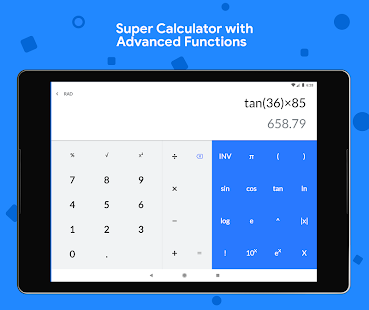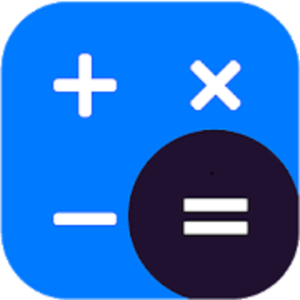Calculator Plus is a complete and easy to use multi calculator which allows you to handle all necessary calculations for everyday life. A perfect calculating tool specially built and beautifully designed for you.

Calculator Plus is best utility which helps you to solve daily simple calculations and problems from complex calculations to unit and currency conversions, percentage, equations, areas, volumes, bmi, loan and so on.

It is a perfect tool for students, teachers, engineers, handyman, contractor or just somebody who struggles with math & conversions. Featuring over 80 free calculators and unit converters fully packed in with a scientific calculator and much better than your phone or handheld calculator.

Key Features :
• Standard calculator, Simple or Scientific layout.
• Convert units or currencies in the same app
• Solves your school homeworks instantly
• Over 80 calculators and unit conveters
• Currency converter with 150 currencies (available offline)
• Smart search for faster navigation
• Double tap anywhere in app to open calculator
• Easy and quick navigation across app

Math Tools
• Percentage calculator
• Average calculator – arithmetic, geometric and harmonic means
• Proportion calculator
• Combinations and permutations
• Area / Perimeter calculator for triangle, square rectangle, parallelogram, trapezoid, rhombus, pentagon, hexagon, circle, circle arc and ellipse
• Volume calculator for cube, rect. prism, sq. pyramid, sq. pyramid frustum, cylinder, cone, conical frustum, sphere, spherical cap, spherical segment and ellipsoid
• Equation solver – linear, quadratic and equation system
• Decimal to fraction
• Prime number checker
• Right triangle calculator
• Heron’s formula (solve a triangle knowing the side lengths)
• Circle solver
• GCF and LCM calculator
• Fraction simplifier
• Number base converter
• Random number generator

Unit converters
• Length converter
• Area converter
• Weight converter
• Volume converter
• Speed converter
• Temperature converter
• Time converter
• Angle converter
• Pressure converter
• Force converter
• Energy converter
• Power converter
• Acceleration converter
• Torque converter
• Digital storage converter
• Data rate converter
• Prefix converter
• Image converter
• Charge converter
• Current converter
• Resistance converter
• Conductance converter
• Inductance converter
• Capacitance converter
• Fuel economy converter
• Cooking converter
• Frequency converter
• Illuminance converter

More
• Body mass index – BMI
• Daily calories burn
• Body fat percentage
• Sales Tax calculator
• Tip calculator
• Loan / EMI calculator
• Smoking cost calculator
• Age calculator
• Elapsed time calculator – years & days, hours & minutes calculator

The app is continuously updated with new features and content. So, stay up to date for new app releases.

What’s New
• New History for Calculator
• New Graphing
• Support for tablets and devices above 4.1
• Bug fixes and performance improvements

Screenshots

•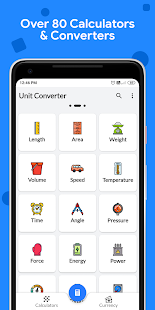•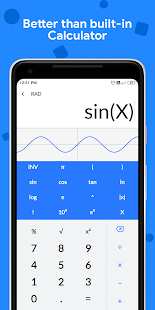•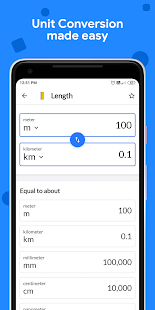•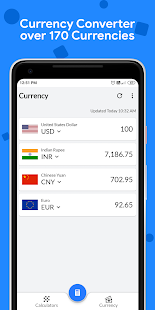•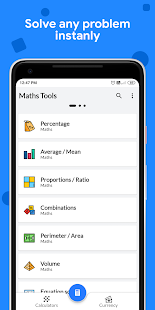•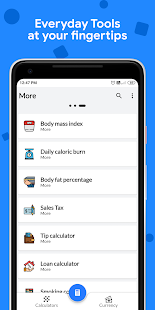•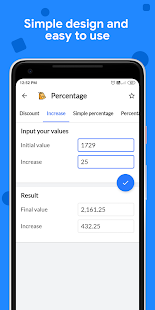•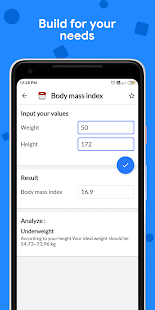•